# Important questions Class 10 Maths Chapter 6 - Triangles

Important questions for Class 10 Maths Chapter 6 Triangles are provided here to help the students in their exam preparation based on the new pattern of CBSE for the 2022-2023 academic session. Students preparing for the board exam are advised to practice these important Triangles questions to score full marks for the questions from this chapter. In the exam, students may come across some of these questions. So, they should not leave any stone unturned for their board exam and must practice these questions. They can also access important questions for 10th maths all chapters at BYJU’S.

The chapter Triangles contains many topics related to a triangle, such as criteria for similarity, congruency, areas of similar triangles and the Pythagoras theorem etc., Students can also get the answers to all the questions in Class 10 Maths of NCERT solutions.

We have provided important questions of this chapter, along with the detailed solutions. After that, we have also provided some questions for students to practice which does not have solutions, and they must solve all of them to gain command over the Triangles topic.

## Important Questions & Answers For Class 10 Maths Chapter 6 Triangles

Q. 1: In the given figure, PS/SQ = PT/TR and ∠ PST = ∠ PRQ. Prove that PQR is an isosceles triangle.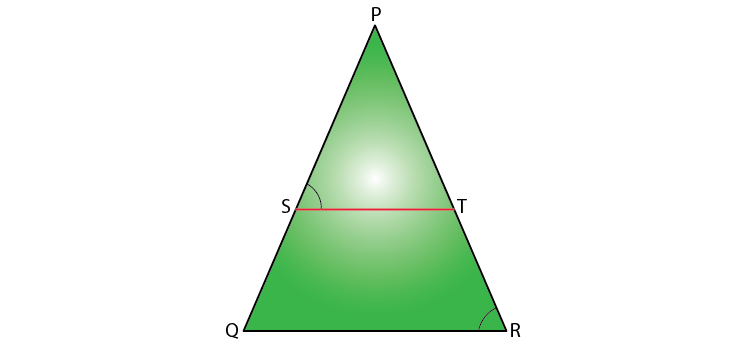Solution:

Given,

PS/SQ = PT/TR

We know that if a line divides any two sides of a triangle in the same ratio, then the line is parallel to the third side.

Therefore, ST // QR

And ∠ PST = ∠ PQR (Corresponding angles) ……..(i)

Also, given,

∠ PST = ∠ PRQ………(ii)

From (i) and (ii),

∠ PRQ = ∠ PQR

Therefore, PQ = PR (sides opposite the equal angles)

Hence, PQR is an isosceles triangle.

Q. 2: In the figure, DE // AC and DF // AE. Prove that BF/FE = BE/EC.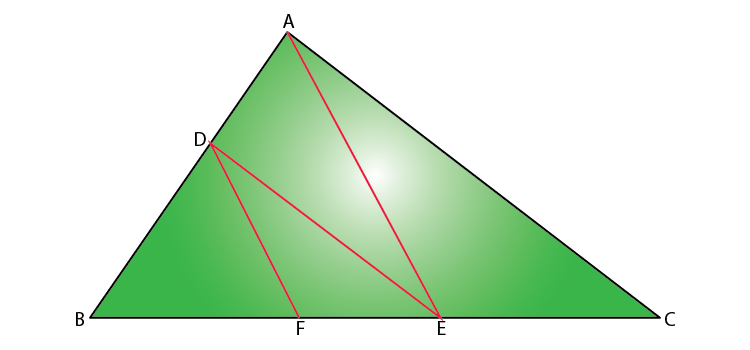Solution:

Given that,

In triangle ABC, DE // AC.

By Basic Proportionality Theorem,

BD/DA = BE/EC……….(i)

Also, given that DF // AE.

Again by Basic Proportionality Theorem,

BD/DA = BF/FE……….(ii)

From (i) and (ii),

BE/EC = BF/FE

Hence proved.

Q. 3: In the given figure, altitudes AD and CE of ∆ ABC intersect each other at the point P. Show that:

(i) ∆AEP ~ ∆ CDP

(ii) ∆ABD ~ ∆ CBE

(iv) ∆ PDC ~ ∆ BEC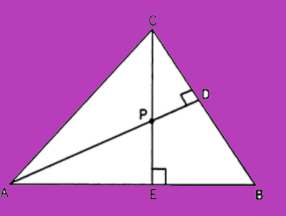Solution:

Given that AD and CE are the altitudes of triangle ABC and these altitudes intersect each other at P.

(i) In ΔAEP and ΔCDP,

∠AEP = ∠CDP (90° each)

∠APE = ∠CPD (Vertically opposite angles)

Hence, by AA similarity criterion,

ΔAEP ~ ΔCDP

(ii) In ΔABD and ΔCBE,

∠ADB = ∠CEB ( 90° each)

∠ABD = ∠CBE (Common Angles)

Hence, by AA similarity criterion,

ΔABD ~ ΔCBE

∠PAE = ∠DAB (Common Angles)

Hence, by AA similarity criterion,

(iv) In ΔPDC and ΔBEC,

∠PDC = ∠BEC (90° each)

∠PCD = ∠BCE (Common angles)

Hence, by AA similarity criterion,

ΔPDC ~ ΔBEC

Q. 4: A vertical pole of length 6 m casts a shadow 4 m long on the ground and at the same time a tower casts a shadow 28 m long. Find the height of the tower

Solution:

Given,

Length of the vertical pole = 6 m

Shadow of the pole = 4 m

Let the height of the tower be h m.

Length of the shadow of the tower = 28 m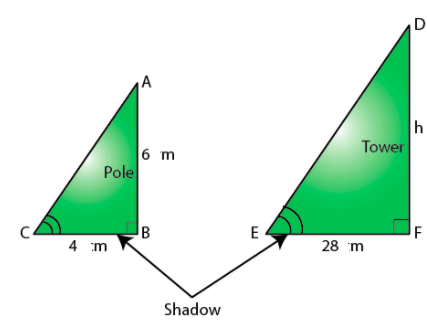In ΔABC and ΔDFE,

∠C = ∠E (angle of elevation)

∠B = ∠F = 90°

By AA similarity criterion,

ΔABC ~ ΔDFE

We know that the corresponding sides of two similar triangles are proportional.

AB/DF = BC/EF

6/h = 4/28

h = (6 ×28)/4

h = 6 × 7

h = 42

Hence, the height of the tower = 42 m.

Q. 5: If ΔABC ~ ΔQRP, ar (ΔABC) / ar (ΔPQR) =9/4 , AB = 18 cm and BC = 15 cm, then find PR.

Solution:

Given that ΔABC ~ ΔQRP.

ar (ΔABC) / ar (ΔQRP) =9/4

AB = 18 cm and BC = 15 cm

We know that the ratio of the areas of two similar triangles is equal to the square of the ratio of their corresponding sides.

ar (ΔABC) / ar (ΔQRP) = BC2/RP2

9/4 = (15)2/RP2

RP2 = (4/9) × 225

PR2 = 100

Therefore, PR = 10 cm

Q. 6: If the areas of two similar triangles are equal, prove that they are congruent.

Solution:

Let ΔABC and ΔPQR be the two similar triangles with equal area.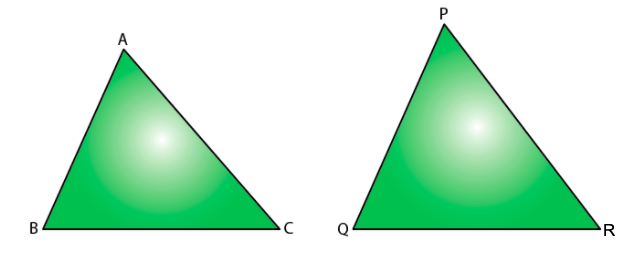To prove ΔABC ≅ ΔPQR.

Proof:

ΔABC ~ ΔPQR

∴ Area of (ΔABC)/Area of (ΔPQR) = BC2/QR2 = AB2/PQ2 = AC2/PR2
⇒ BC2/QR2 = AB2/PQ2 = AC2/PR2 = 1 [Since, ar (ΔABC) = ar (ΔPQR)] ⇒ BC2/QR2 = 1
⇒ AB2/PQ2 = 1
⇒ AC2/PR2 = 1
BC = QR
AB = PQ
AC = PR
Therefore, ΔABC ≅ ΔPQR [SSS criterion of congruence]

Q. 7: O is any point inside a rectangle ABCD as shown in the figure. Prove that OB2 + OD2 = OA2 + OC2.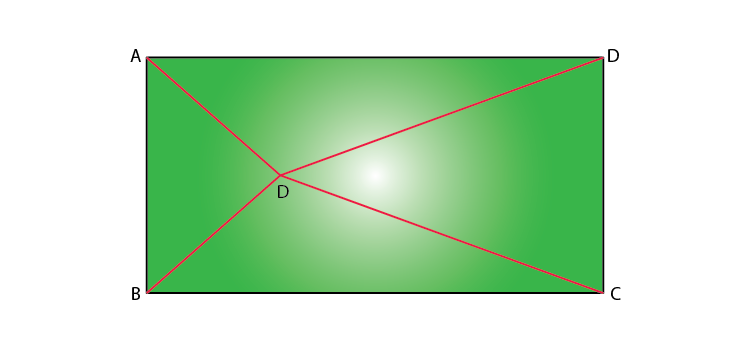Solution:

Through O, draw PQ || BC so that P lies on AB and Q lies on DC.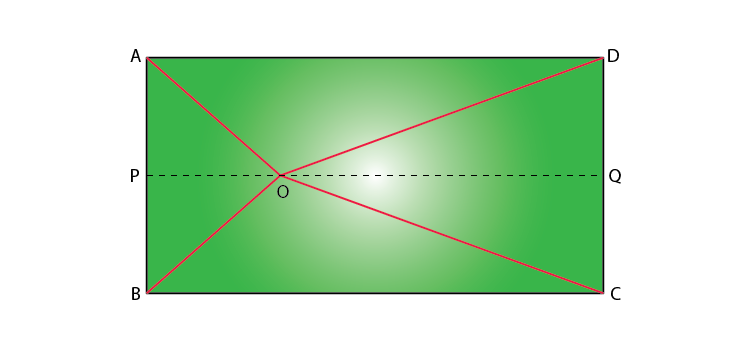PQ || BC

Therefore, PQ ⊥ AB and PQ ⊥ DC (∠ B = 90° and ∠ C = 90°)

So, ∠ BPQ = 90° and ∠ CQP = 90°

Hence, BPQC and APQD are both rectangles.

By Pythagoras theorem,

In ∆ OPB,

OB2 = BP2 + OP2…..(1)

Similarly,

In ∆ OQD,

OD2 = OQ2 + DQ2…..(2)

In ∆ OQC,

OC2 = OQ2 + CQ2…..(3)

In ∆ OAP,

OA2 = AP2 + OP2…..(4)

OB2+ OD2 = BP2 + OP2 + OQ2 + DQ2

= CQ2 + OP2 + OQ2 + AP2

(since BP = CQ and DQ = AP)

= CQ2 + OQ2 + OP2 + AP2

= OC2 + OA2 [From (3) and (4)]

Hence proved that OB2 + OD2 = OA2 + OC2.

Q. 8: Sides of triangles are given below. Determine which of them are right triangles.

In case of a right triangle, write the length of its hypotenuse.

(i) 7 cm, 24 cm, 25 cm

(ii) 3 cm, 8 cm, 6 cm

Solution:

(i) Given, sides of the triangle are 7 cm, 24 cm, and 25 cm.

Squaring the lengths of the sides of the, we will get 49, 576, and 625.

49 + 576 = 625

(7)2 + (24)2 = (25)2

Therefore, the above equation satisfies the Pythagoras theorem. Hence, it is a right-angled triangle.

Length of Hypotenuse = 25 cm

(ii) Given, sides of the triangle are 3 cm, 8 cm, and 6 cm.

Squaring the lengths of these sides, we will get 9, 64, and 36.

Clearly, 9 + 36 ≠ 64

Or, 32 + 62 ≠ 82

Therefore, the sum of the squares of the lengths of two sides is not equal to the square of the length of the hypotenuse.

Hence, the given triangle does not satisfy the Pythagoras theorem.

Q.9: If a line is drawn parallel to one side of a triangle to intersect the other two sides in distinct points, then prove that the other two sides are divided in the same ratio.

Solution:

Consider a triangle ΔABCand draw a line PQ parallel to the side BC of ΔABC and intersect the sides AB and AC in P and Q, respectively.

To prove: AP/PB = AQ/QC

Cosntruction:

Join the vertex B of ΔABC to Q and the vertex C to P to form the lines BQ and CP and then drop a perpendicular QN to the side AB and draw PM⊥AC as shown in the given figure.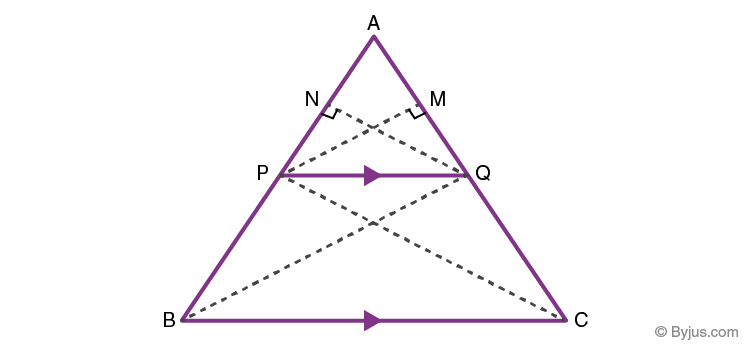Proof:

Now the area of ∆APQ = 1/2 × AP × QN (Since, area of a triangle= 1/2× Base × Height)

Similarly, area of ∆PBQ= 1/2 × PB × QN

area of ∆APQ = 1/2 × AQ × PM

Also,area of ∆QCP = 1/2 × QC × PM ………… (1)

Now, if we find the ratio of the area of triangles ∆APQand ∆PBQ, we have

$$\begin{array}{l}\frac {area ~of~ \triangle APQ}{area~ of~ \triangle PBQ} = \frac {\frac 12 ~\times ~AP~\times ~QN}{ \frac 12~\times ~PB~\times ~QN} = \frac {AP}{PB}\end{array}$$
$$\begin{array}{l}Similarly,\ \frac {area~ of~ \triangle APQ}{area~ of~ \triangle QCP} = \frac {\frac12~ \times ~ AQ~\times ~PM}{\frac 12 ~\times ~QC~\times ~PM} = \frac {AQ}{QC} …(2)\end{array}$$

According to the property of triangles, the triangles drawn between the same parallel lines and on the same base have equal areas.

Therefore, we can say that ∆PBQ and QCP have the same area.

area of ∆PBQ = area of ∆QCP …………..(3)

Therefore, from the equations (1), (2) and (3), we can say that,

AP/PB = AQ/QC

Q.10: In the figure, DE || BC. Find the length of side AD, given that AE = 1.8 cm, BD = 7.2 cm and CE = 5.4 cm.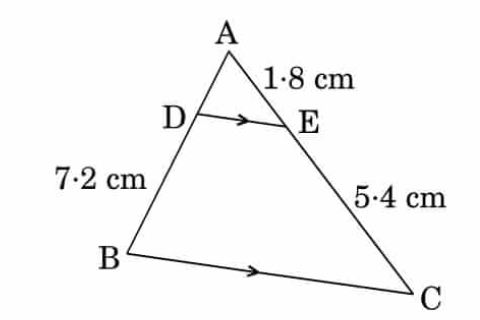Solution:

Given,

DE || BC

AE = 1.8 cm, BD = 7.2 cm and CE = 5.4 cm

By basic proportionality theorem,

= 7.2/4

= 2.4

Q.11: Given ΔABC ~ ΔPQR, if AB/PQ = ⅓, then find (ar ΔABC)/(ar ΔPQR).

Solution:

Given,

ΔABC ~ ΔPQR

And

AB/PQ = ⅓,

We know that The ratio of the areas of two similar triangles is equal to the square of the ratio of their corresponding sides.

(ar ΔABC)/(ar ΔPQR) = AB2/PQ2 = (AB/PQ)2 = (⅓)2 = 1/9

Therefore, (ar ΔABC)/(ar ΔPQR) = 1/9

Or

(ar ΔABC) : (ar ΔPQR) = 1 : 9

Q.12: The sides of two similar triangles are in the ratio 7 : 10.  Find the ratio of areas of these triangles.

Solution:

Given,

The ratio of sides of two similar triangles = 7 : 10

We know that the ratio of the areas of two similar triangles is equal to the square of the ratio of their corresponding sides.

The ratio of areas of these triangles = (Ratio of sides of two similar triangles)2

= (7)2 : (10)2

= 49 : 100

Therefore, the ratio of areas of the given similar triangles is 49 : 100.

Q.13: In an equilateral ΔABC, D is a point on side BC such that BD = (⅓) BC. Prove that 9(AD)2 = 7(AB)2.

Solution:

Given, ABC is an equilateral triangle.

And D is a point on side BC such that BD = (1/3)BC.

Let a be the side of the equilateral triangle and AE be the altitude of ΔABC.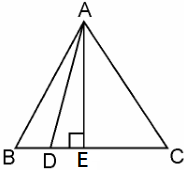∴ BE = EC = BC/2 = a/2

And, AE = a√3/2

Given, BD = 1/3BC

∴ BD = a/3

DE = BE – BD = a/2 – a/3 = a/6

= [(a√3)/2]2 + (a/6)2

= (3a2/4) + (a2/36)

= (37a2 + a2)/36

= (28a2)/36

= (7/9)a2

= (7/9) (AB)2

Q.14: Prove that in a right triangle, the square of the hypotenuse is equal to the sum of the squares of the other two sides.

Solution:

Given: A right-angled triangle ABC, right-angled at B.

To Prove: AC2 = AB2 + BC2

Construction: Draw a perpendicular BD meeting AC at D.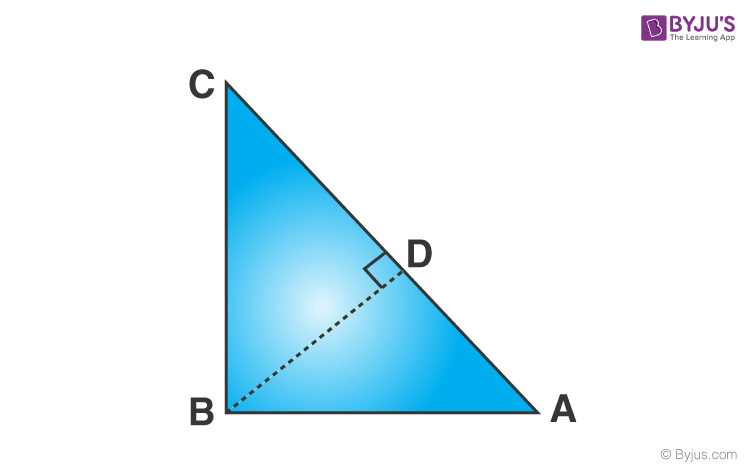Proof:

∠A = ∠A → common

Using the AA criterion for the similarity of triangles,

⇒ AB2 = AC x AD ……(1)

Considering  ΔABC and ΔBDC from the figure.

C = ∠C  → common

∠CDB = ∠ABC = 90°

Using the Angle Angle(AA) criterion for the similarity of triangles, we conclude that,

ΔBDC ~ ΔABC

Therefore, CD/BC = BC/AC

⇒ BC2 = AC x CD …..(2)

By adding equation (1) and equation (2), we get:

AB2 + BC2 = (AC x AD) + (AC x CD)

AB2 + BC2 = AC (AD + CD) …..(3)

AB2 + BC2 = AC (AC) {since AD + CD = AC}

AB2 + BC2 = AC2

Hence proved.

Q.15: In the figure, if PQ || RS, prove that ∆ POQ ~ ∆ SOR.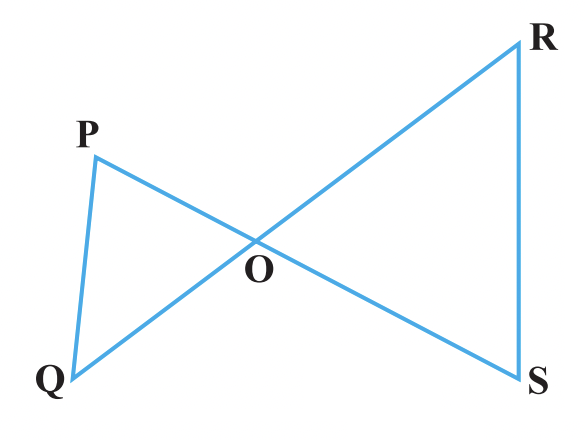Solution:

Given,

PQ || RS

∠P = ∠S (Alternate angles)

and ∠Q = ∠R

Also, ∠POQ = ∠SOR (Vertically opposite angles)

Therefore, ∆ POQ ~ ∆ SOR (by AAA similarity criterion)

Hence proved.

## Video Lesson on BPT and Similar Triangles### Class 10 Maths Chapter 6 Triangles Questions for Practice

1. Prove that the ratio of the areas of two similar triangles is equal to the square of the ratio of their corresponding medians.
2. Two right triangles ABC and DBC are drawn on the same hypotenuse BC and on the same side of BC. If AC and BD intersect at P, prove that AP × PC = BP × DP.
3. Diagonals of a trapezium PQRS intersect each other at the point O, PQ || RS and PQ = 3RS. Find the ratio of the areas of triangles POQ and ROS.
4. Prove that the area of an equilateral triangle described on one side of the square is equal to half the area of the equilateral triangle described on one of its diagonal.
5. Prove that the ratio of the areas of two similar triangles is equal to the square of the ratio of their corresponding sides.
6. Corresponding sides of two similar triangles are in the ratio of 2 : 3. If the area of the smaller triangle is 48 cm2, find the area of the larger triangle.
7. A foot of a 10 m long ladder leaning against a vertical wall is 6 m away from the base of the wall. Find the height of the point on the wall where the top of the ladder reaches.
8. An aeroplane leaves an airport and flies due north at a speed of 1000 km per hour. At the same time, another aeroplane leaves the same airport and flies due west at a speed of 1200 km per hour. How far apart will be the two planes after 1 1/2 hours?
9. Diagonals AC and BD of a trapezium ABCD with AB || DC intersect each other at the point O. Using a similarity criterion for two triangles, show that OA/OC = OB/OD.
10. Prove that if in a triangle square on one side is equal to the sum of the squares on the other two sides, then the angle opposite the first side is a right angle.